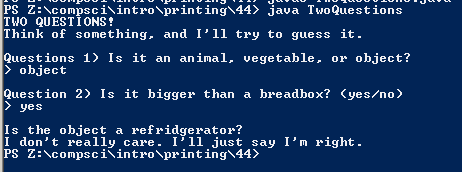PROGRAMMING AND CODE

# Assignemnt #44

## Code

```/// Name: Justin Li
/// Period 7
/// Program Name:  TwoQuestions
/// File Name: TwoQuestions.java
/// Date Finished: 11/13/15

import java.util.Scanner;

public class TwoQuestions {

public static void main(String[] args) {

Scanner keyboard = new Scanner(System.in);

String q1, q2, guess;

System.out.println("TWO QUESTIONS!");
System.out.println("Think of something, and I'll try to guess it.");

System.out.println();

System.out.println("Questions 1) Is it an animal, vegetable, or object?");
System.out.print("> ");
q1 = keyboard.next();

System.out.println();

System.out.println("Question 2) Is it bigger than a breadbox? (yes/no)");
System.out.print("> ");
q2 = keyboard.next();

System.out.println();

if (q1.equals("animal")) {
if (q2.equals("yes")) {
guess = "an elephant";
}
else if (q2.equals("no")) {
guess = "a mouse";
}
else {
System.out.println("You didn't give a correct answer for Question 2.");
guess = "N/A";
}
}
else if (q1.equals("vegetable")) {
if (q2.equals("yes")) {
guess = "a pumpkin";
}
else if (q2.equals("no")) {
guess = "a tomato";
}
else {
System.out.println("You didn't give a correct answer for Question 2.");
guess = "N/A";
}
}
else if (q1.equals("object")) {
if (q2.equals("yes")) {
guess = "a refridgerator";
}
else if (q2.equals("no")) {
guess = "a watch";
}
else {
System.out.println("You didn't give a correct answer for Question 2.");
guess = "N/A";
}
}
else {
System.out.println("You didn't give a correct answer for Question 1.");
guess = "N/A";
}

if (guess.equals("N/A")) {